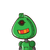# The arithmetic mean of 8 observations was found to be 12.5, then the sum of the observations is ___a)100b)

The arithmetic mean of 8 observations was found to be 12.5, then the sum of the observations is ___

a)100

b)120

c)130

d)140​

### 1 thought on “The arithmetic mean of 8 observations was found to be 12.5, then the sum of the observations is ___<br /><br />a)100<br /><br />b)”

1.### Solution:–

Arithmetic Mean of Observations = 12.5

Number of Observations = 8

✯ Arithmetic Mean = Sum of Observations÷Number of Observations

✯ Sum of Observations = Mean×Number of Observations

→ 12.5×8

→ 100

Sum of Observations = Option a. 100

[tex]\Large{✓}[/tex]

Hope It Helps You ✌️